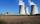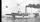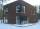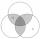# Algebra - math word problems

#### Number of problems found: 2648

• Electricity consumption costLast year Karol family reduced its electricity consumption by 31% compared to the previous year and paid CZK 2883 less. How many CZK is electricity last year and how many two year ago?
• Trapezoid thirdsThe ABCD trapezoid with the parallel sides of the AB and the CD and the E point of the AB side if the segment DE divides the trapezoid into two parts with the same area. Find the length of the AE line segment.
• Trapezoid 15Area of trapezoid is 266. What value is x if bases b1 is 2x-3, b2 is 2x+1 and height h is x+4
• Have solutionThe sum of four consecutive even numbers is 96. Determine these numbers.
• SteamerAt 6 hours 40 minutes steamer sailed from the port at speed 12 km/h. At exactly 10 hours started sail motorboat at speed 42 km/h. When motorboat will catch steamer?
• Real estateResidential house has three entrances numbered by odd numbers arithmetic progression. The sum of the two numbers on the corner entrances is 50. Calculate the highest of this three numbers.
• Isosceles right triangleContents of an isosceles right triangle is 18 dm2. Calculate the length of its base.
• RectangleFind the dimensions of the rectangle, whose perimeter is 108 cm and the length is 25% larger than the width.
• CubesCarol with cut bar 12 cm x 12 cm x 135 cm to the cubes. Find the sum of all the surfaces of the resulting cubes.
• Cube-shaped boxDesign the size of the smallest possible cube-shaped box where three types of 3cm, 5cm, 6cm small cubes could be stacked to make full use of the box space (each type of cube separately). Can you find out how many smallest cubes are in the box?
• Potatoes 6The farmer brought potatoes to the market. In the first hour he sold two-fifths of the potatoes brought, in the second hour he sold five-sixth of the remaining potatoes, and in the third hour he had sold the last 40kg of potatoes. How many kg of potatoes
• Equilateral triangleThe equilateral triangle has a 23 cm long side. Calculate its content area.
• TreesAlong the road were planted 250 trees of two types. Cherry for 60 CZK apiece and apple 50 CZK apiece. The entire plantation cost 12,800 CZK. How many was cherries and apples?
• MO Z6-6-1Write integers greater than 1 to the blanks in the following figure, so that each darker box was product of the numbers in the neighboring lighter boxes. What number is in the middle box?
• BenchesThe park has 64 benches. Occupied are by 18 more than empty. How many benches are occupied and empty ?
• BillboardRectangular billboard is 2.5 m long with a diagonal 2.8 m long. Calculate the perimeter and the content area of the billboard.
• Isosceles triangleCalculate area and perimeter of an isosceles triangle ABC with base AB if a = 6 cm, c = 7 cm.
• RhombusIf we increase the rhombus side by 2.4 cm; its perimeter is 20 cm. How long was the side of the rhombus?
• Land - isosceles trapezoidCalculate the content and perimeter of the building plot in the form of an isosceles trapezoid with bases 120m, 95m and height 50m.
• Empty canisterThe canister is filled with oil weight 17 kg. If filled only half weight 9 kg. What weight does my empty canister have?

Do you have an interesting mathematical word problem that you can't solve it? Submit a math problem, and we can try to solve it.

We will send a solution to your e-mail address. Solved examples are also published here. Please enter the e-mail correctly and check whether you don't have a full mailbox.

Please do not submit problems from current active competitions such as Mathematical Olympiad, correspondence seminars etc...